# My First Arduino 101 Car Controlled by BLE

Recently I received an Arduino 101 kit from Hackster. My joy was great because it had a lot to do new projects!

BeginnerFull instructions provided1 hour2,823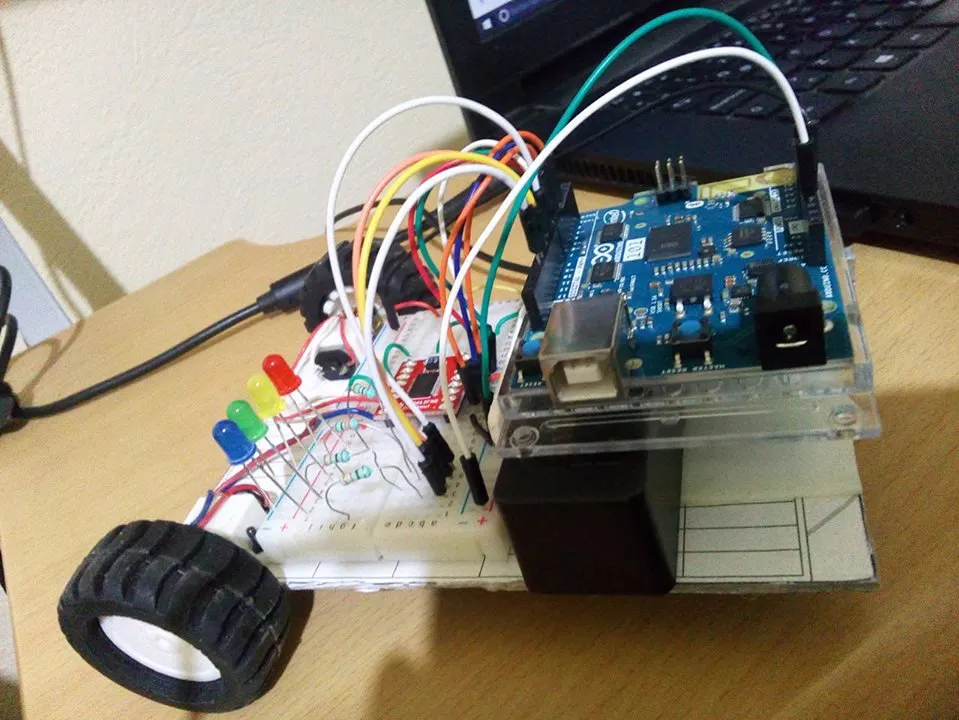## Things used in this project

### Hardware components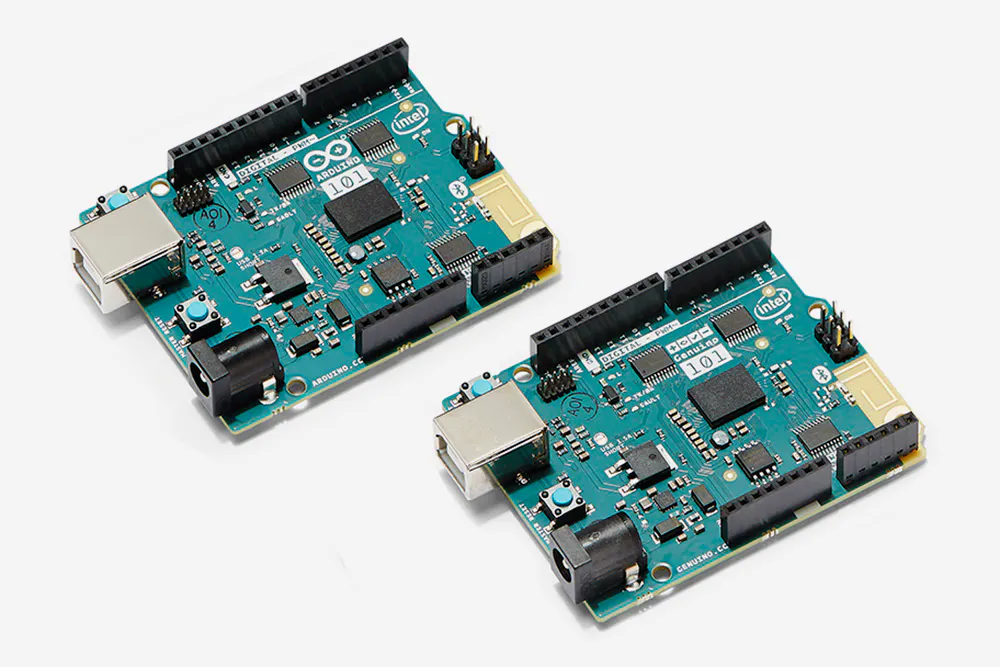Arduino 101 & Genuino 101
×1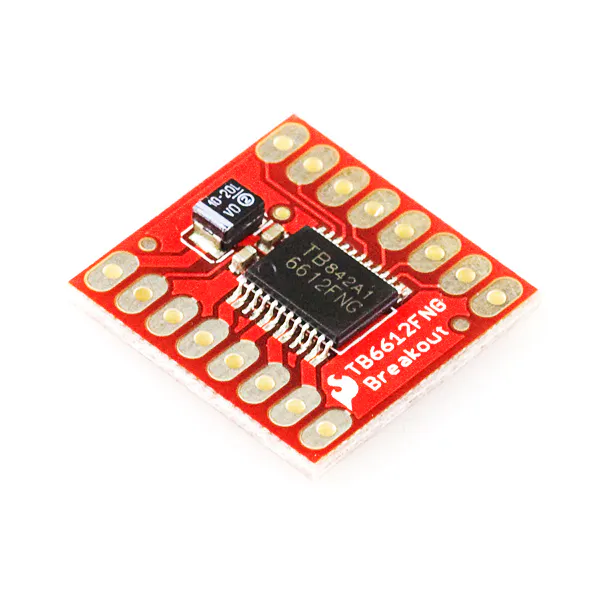SparkFun Motor Driver - Dual TB6612FNG (1A)
×1×1Jumper wires (generic)
×1
 DC motor (generic)
×2LED (generic)
×4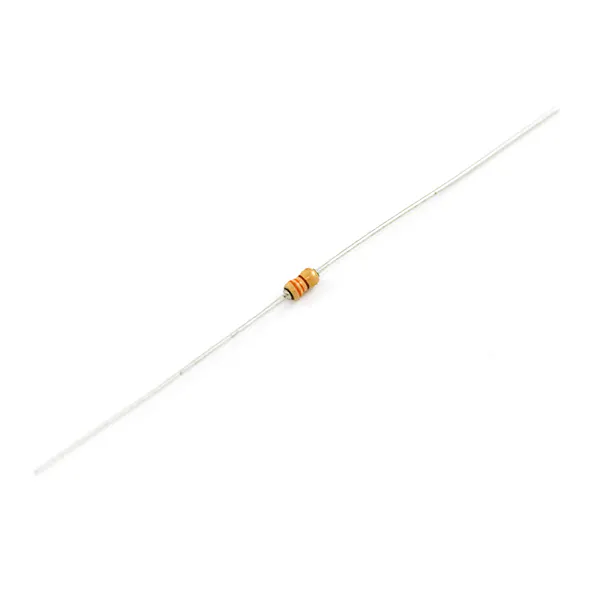Resistor 330 ohm
×4

## Schematics

### Car diagram

Easy diagram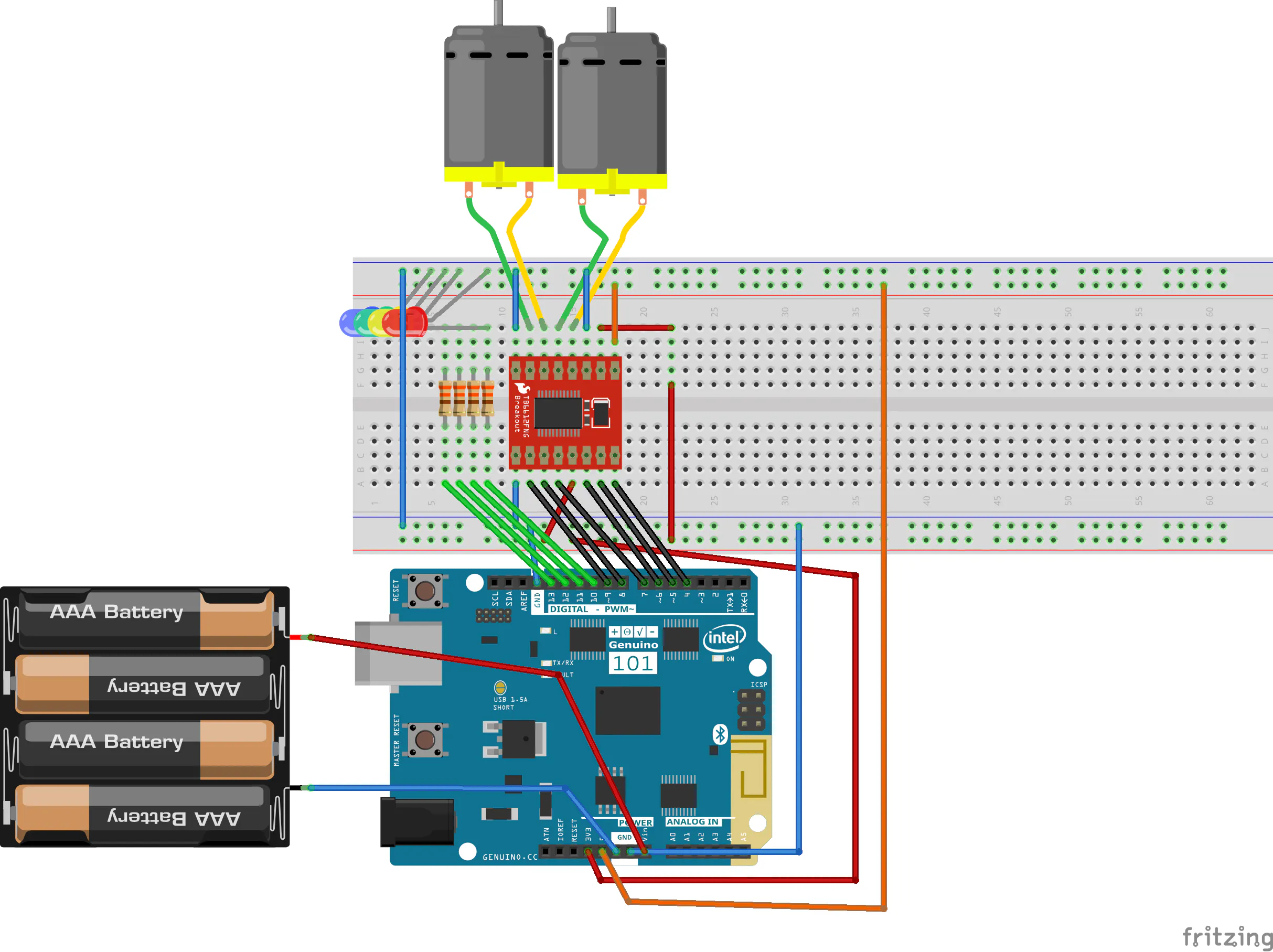## Code

### Arduino car code

Arduino
```#define BLYNK_PRINT Serial
#include <BlynkSimpleCurieBLE.h>
#include <CurieBLE.h>

char auth[] = "5733e53afff240d79e49d7acc0849faa";

BLEPeripheral  blePeripheral;

const int DIR_A1 = 4;
const int DIR_B1 = 5;
const int PWM1 = 6;
const int DIR_A2 = 7;
const int DIR_B2 = 8;
const int PWM2 = 9;
const int luz = 13;
int pinValue1=0,pinValue2=0,pinValue3=0,pinValue4=0;
bool cambio=LOW;
byte random1;
const byte pin1=10,pin2=11,pin3=12,pin4=13;
long currentMillis;
long previousMillis = 0;

BLYNK_WRITE(V1)
{
pinValue1 = param.asInt(); // assigning incoming value from pin V1 to a variable

}
BLYNK_WRITE(V2)
{
pinValue2 = param.asInt(); // assigning incoming value from pin V1 to a variable

}
BLYNK_WRITE(V3)
{
pinValue3 = param.asInt(); // assigning incoming value from pin V1 to a variable

}
BLYNK_WRITE(V4)
{
pinValue4 = param.asInt(); // assigning incoming value from pin V1 to a variable

}

void setup()
{
// Debug console
Serial.begin(9600);
pinMode(DIR_A1, OUTPUT);
pinMode(DIR_B1, OUTPUT);
pinMode(PWM1, OUTPUT);
pinMode(DIR_A2, OUTPUT);
pinMode(DIR_B2, OUTPUT);
pinMode(PWM2, OUTPUT);
pinMode(luz, OUTPUT);
pinMode(pin1,OUTPUT);
pinMode(pin2,OUTPUT);
pinMode(pin3,OUTPUT);
pinMode(pin4,OUTPUT);
delay(1000);

blePeripheral.setLocalName("Blynk");
blePeripheral.setDeviceName("Blynk");
blePeripheral.setAppearance(384);

Blynk.begin(blePeripheral, auth);

blePeripheral.begin();

Serial.println("Waiting for connections...");

}

void loop()
{
blePeripheral.poll();
Blynk.run();
digitalWrite(luz, HIGH);
if(pinValue1==1)
{
digitalWrite(DIR_A1, LOW);
digitalWrite(DIR_B1, HIGH);
digitalWrite(DIR_A2, LOW);
digitalWrite(DIR_B2, HIGH);
analogWrite(PWM1, 255);
analogWrite(PWM2, 255);
}
else if(pinValue2==1)
{
cambio=HIGH;
digitalWrite(DIR_A1, HIGH);
digitalWrite(DIR_B1, LOW);
digitalWrite(DIR_A2, HIGH);
digitalWrite(DIR_B2, LOW);
analogWrite(PWM1, 255);
analogWrite(PWM2, 255);
}

else if(pinValue3==1)
{
cambio=HIGH;
digitalWrite(DIR_A1, HIGH);
digitalWrite(DIR_B1, LOW);
digitalWrite(DIR_A2, LOW);
digitalWrite(DIR_B2, HIGH);
analogWrite(PWM1, 100);
analogWrite(PWM2, 255);
}

else if(pinValue4==1)
{
cambio=HIGH;
digitalWrite(DIR_A1, LOW);
digitalWrite(DIR_B1, HIGH);
digitalWrite(DIR_A2, HIGH);
digitalWrite(DIR_B2, LOW);
analogWrite(PWM1, 255);
analogWrite(PWM2, 100);
}

else if(pinValue1==0 && pinValue2==0 && pinValue3==0 && pinValue4==0 )
{
digitalWrite(DIR_A1, HIGH);
digitalWrite(DIR_B1, LOW);
digitalWrite(DIR_A2, HIGH);
digitalWrite(DIR_B2, LOW);
analogWrite(PWM1, 0);
analogWrite(PWM2, 0);
}
random1 = random(10,14);
currentMillis = millis();
// if 10s have passed, check the sensor:
if (currentMillis - previousMillis >= 500) {
previousMillis = currentMillis;
digitalWrite(random1, estado) ;            // escribimos el nuevo valor
}

}
```

## Credits

### Alexis Santiago Allende

0 projects • 56 followers
Im a person who since young feel a passion for electronics, I also like to cook pizza and travel. Now Im working on the internet of things